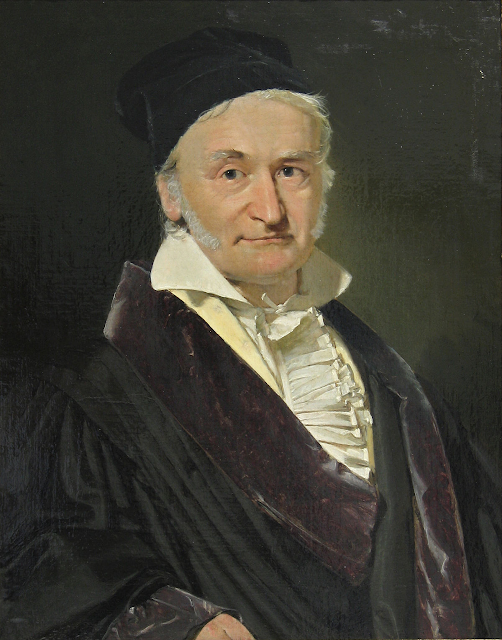## 2022年4月16日 星期六

### 高斯的復活節演算法

原來根據聖經記載，耶穌復活的日子是猶太人的逾越節（即是猶太曆尼散月十四日）後的首個星期日。猶太曆屬於陰曆，尼散月十四日基本上就是春分後的滿月日的前一天。於是羅馬教會用了321日作為春分的估測（春分並不一定在321日，根據今天使用的格里曆曆法，它也可以在322日，根據舊的儒略曆曆法，更加可以差得非常遠）。綜合了以上眾多的考慮，教會最終作出了這規定。發明復活節演算法的數學家哥斯

1. a = 年份 (mod 19) （即年份除以19的餘數，下同）

2. b = 年份 (mod 4)

3. c = 年份 (mod 7)

4. k = 年份 / 100小於或等於年份除以100的最大整，下同）

5. p = (13 + 8) / 256. q = k / 4

7. M = 15 – p + k – q (mod 30)

8. N = 4 + k – q (mod 7)

9. d = 19a + M (mod 30)

10. e = 2b + 4c + 6d + N (mod 7)

11. 復活節的日子是3(22 + d + e)日或4(d + e – 9 )

12. 如果d = 29e = 6，那麼用426日去取代419

13. 如果d = 28e = 611M + 11 (mod 30) < 19，那麼用425日去取代418

1. a = 2022 (mod 19) = 8

2. b = 2022 (mod 4) = 2

3. c = 2022 (mod 7) = 6

4. k = 2022 / 100 = 20

5. p = (13 + 8 x 20) / 25 = 6

6. q = 20 / 4 = 5

7. M = 15 – 6 + 20 – 5 (mod 30) = 24

8. N = 4 + k – q (mod 7) = 5

9. d = 19(8) + 24 (mod 30) = 26

10. e = 2(2) + 4(6) + 6(26) + 5 (mod 7) = 0

11. 因此復活節的日子是3(22 + 26 + 0)日或4(26 + 0 – 9 )日，即348日或417日。348日當然是不可能的，那復活節的日期應是417日。這也與我們的認知相符。